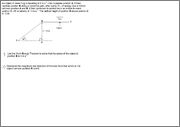# Inclined plane, Calculate friction force

TheRedDevil18

## Homework StatementWnet = Ek

## The Attempt at a Solution

1) That was fine.
2) I just want to confirm if this answer is right?, I got 12.28N. Used work energy theorem.

Sorry for double post, edited the title

Homework Helper
Gold Member
2022 Award

## Homework StatementWnet = Ek

## The Attempt at a Solution

1) That was fine.
2) I just want to confirm if this answer is right?, I got 12.28N. Used work energy theorem.

Sorry for double post, edited the title

Seems a bit high. Pls post your working.

TheRedDevil18
Seems a bit high. Pls post your working.

Sorry my mistake, forgot something in my calculation. Anyway here's my working

Wnet = Ek
Wg + Wf + Wn = 1/2*m(vf^2 - vi^2)
(5*9.8*sin30*20) - 20Ff + 0 = 1/2*5(12^2 - 6^2)...That 20 is the length of the incline
Ff = 11N...Now correct?

Homework Helper
Gold Member
2022 Award
Sorry my mistake, forgot something in my calculation. Anyway here's my working

Wnet = Ek
Wg + Wf + Wn = 1/2*m(vf^2 - vi^2)
(5*9.8*sin30*20) - 20Ff + 0 = 1/2*5(12^2 - 6^2)...That 20 is the length of the incline
Ff = 11N...Now correct?

Yes, that looks right.

•1 person